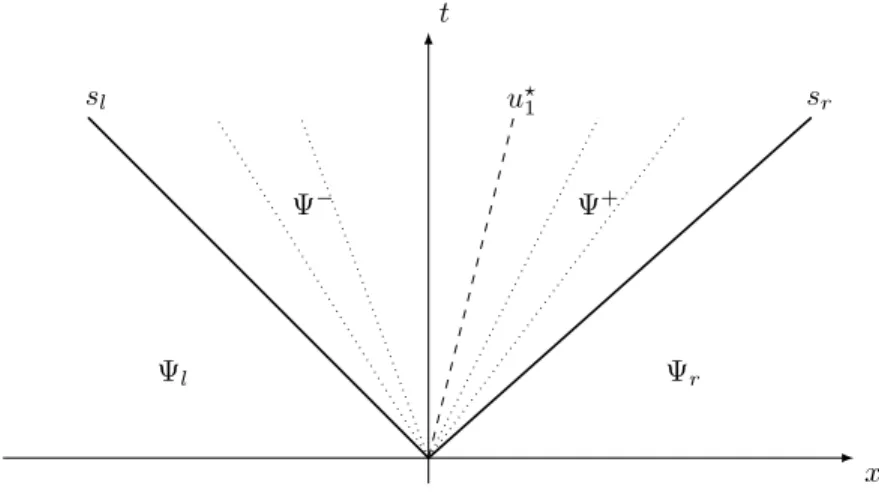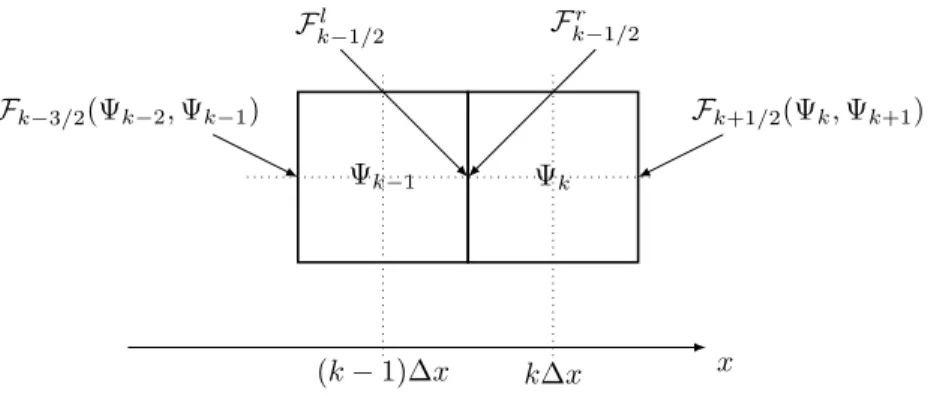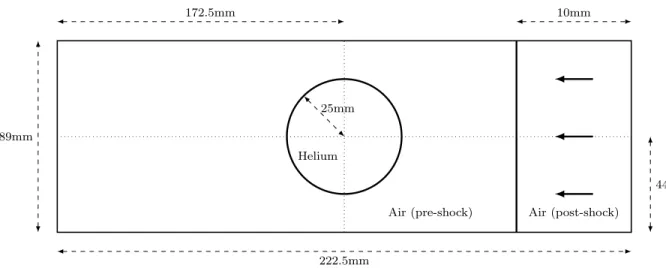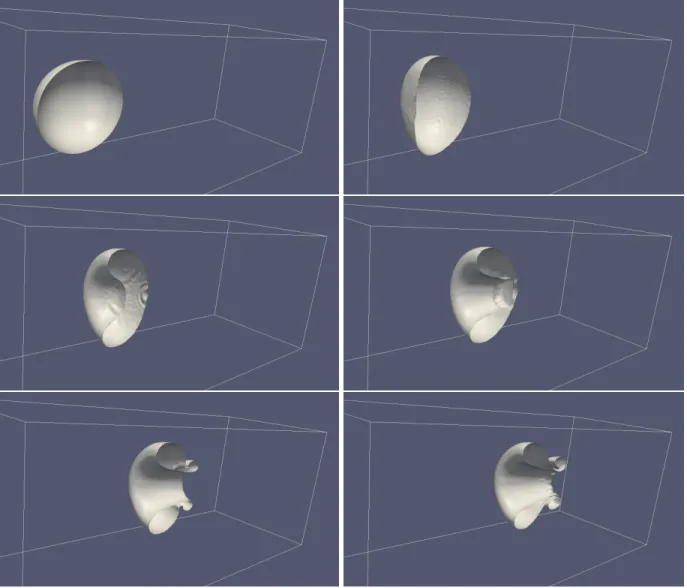• Aucun résultat trouvé

# Eulerian Scheme for 3D multimaterials

N/A
N/A
Protected

Partager "Eulerian Scheme for 3D multimaterials"

Copied!
13
0
0

Texte intégral

(1)

### Technology researchers and makes it freely available over the web where possible.

This is an author-deposited version published in: https://sam.ensam.eu

Handle ID: .http://hdl.handle.net/10985/9038

To cite this version :

Alexia BRAUER (DE), Angelo IOLLO, Thomas MILCENT - Eulerian Scheme for 3D multimaterials - In: Eighth International Conference on Computational Fluid Dynamics (ICCFD8(8; 2014 ; Chine), China, 201407 Eighth International Conference on Computational Fluid Dynamics (ICCFD8) -2014

(2)

Eighth International Conference on

Computational Fluid Dynamics (ICCFD8), Chengdu, China, July 14-18, 2014

ICCFD8-2014-0393

## Eulerian Scheme for 3D multimaterials

1

1

2

1

2

### Corresponding author: angelo.iollo@math.u-bordeaux1.fr

Abstract: This paper is devoted to the 3D extension of a sharp interface multimaterial method. We present preliminary results for 3D stiff cases of shock air/helium/water interaction and impacts of projectiles on elastic bodies immersed in air.

Keywords: Compressible multimaterial, Cartesian mesh, Eulerian elasticity, HLLC Riemann Solver.

### Introduction

Physical and engineering problems that involve several materials are ubiquitous in nature and in applications. The main contributions in the direction of simulating these phenomena go back to . We have already developed a numerical scheme to solve the Eulerian equations for multimaterial (see  and  for 1D numerical results and  for 2D results). This scheme is based on a directional splitting on a fixed Cartesian mesh where the fluxes are computed by a HLLC approximate Riemann solver. This paper is devoted to the extension of this method in 3D, where two additional elastic waves corresponding to a torsion perturbation are present.

### The Eulerian Model

The conservative form of elastic media equations in the Eulerian framework are       ρt+ divx(ρu) = 0 (ρu)t+ divx(ρu ⊗ u − σ) = 0 (∇xY )t+ ∇x(u · ∇xY ) = 0

(3)

B(x, t) = [∇xY (x, t)]−1[∇xY (x, t)]−T/J (x, t)2/3 J (x, t) = det([∇xY (x, t)])−1 (3) The constants γ, a, b, p∞, ρ0, have the usual meaning and χ is the shear modulus. The two first terms of (2) represent a Van der Waals gas, the third one a stiffened gas and the last one represent a Neo-Hookean elastic solid. The stress tensor σ is then derived from this constitutive law

σ = −p(ρ, s)I + 2χJ−1  B −Tr(B) 3 I  (4) where the pressure p is given by

p(ρ, s) = −p∞− aρ2+ κ(s)  1 ρ− b −γ (5)

### Numerical scheme

Let x = (x1, x2, x3) be the coordinates in the canonical basis of R3, u = (u1, u2, u3) the velocity components, Y = (Y1, Y2, Y3) the components of Y and σij the components of the stress tensor σ. Also, let us denote by , k the differentiation with respect to xk. Our scheme is based on a directional splitting on a cartesian mesh. When computing the numerical fluxes at cell interfaces in the x1direction we have (Y,2i)t= (Y,3i)t= 0 thus Yi

,2and Y,3i are constants. The governing equations in conservative form in the x1direction become

Ψt+ (F (Ψ)),1= 0 (6)

with φi= ρuiand ψ = ρe

Ψ =                   ρ φ1 φ2 φ3 Y1 ,1 Y2 ,1 Y3 ,1 ψ                   F (Ψ) =                   φ1 (φ1)2 ρ − σ 11 φ1φ2 ρ − σ 21 φ1φ3 ρ − σ 31 φ1Y,11+φ2Y,21+φ3Y,31 ρ φ1Y,12+φ2Y,22+φ3Y,32 ρ φ1Y,13+φ2Y,23+φ3Y,33 ρ φ1ψ−(σ11φ1+σ21φ2+σ31φ3) ρ                  

The numerical fluxes are computed with a HLLC approximate Riemann solver. This solver requires the analytical expression of the wave velocities which are the eigenvalues of the Jacobian of F (Ψ). The wave velocities are defined locally by infinitesimal variations of the conservative variables. Therefore the energy equation can be replaced by the transport equation on the entropy st+ u · ∇s = 0 and the wave velocity calculations are performed at fixed entropy. We introduce the notation

Σ = [∇σ][∇Y ] :=   σ11 ,1 σ11,2 σ,311 σ,121 σ21,2 σ,321 σ31 ,1 σ31,2 σ,331     Y1 ,1 Y,21 Y,31 Y,12 Y,22 Y,32 Y3 ,1 Y,23 Y,33   (7)

where σ,kij denote the derivative of σij with respect to Yk

,1. The wave speeds are then given by

ΛE=  u1, u1± r α1 ρ, u1± r α2 ρ, u1± r α3 ρ 

(4)

X3+ Tr(Σ)X2+ Tr(Cof((Σ))X + Det(Σ) = 0 (8) Therefore, the conditions for the system (6) to be hyperbolic are α1> 0, α2> 0 and α3> 0.

The numerical flux function F (Ψl, Ψr) is computed using the HLLC  approximate solver modified according to  at the multimaterial interface. The wave pattern involves seven waves in the exact problem, but the HLLC approximate solver approaches the solution using three waves and thus defining two inter-mediate states Ψ− and Ψ+ (see Fig 1). The three waves are the contact discontinuity and the two fastest waves. Rankine Hugoniot conditions are used to determine the two intermediate states.

x t sr u?1 sl Ψr Ψ+ Ψ− Ψl

Figure 1: HLLC solver wave pattern.

The multimaterial solver is detailed in 1D for sake of clarity. In 3D we use exactly the same method in the three directions. We consider a case where the material discontinuity is between the cell centers k − 1 and k. The material discontinuity can separate materials with different constitutive laws or discontinuous initial states of the same material. The main idea of the multimaterial solver is that we take (see Fig 2)

Fl

k−1/2= F

Fr

k−1/2= F

+ (9)

where F−= F (Ψ−) and F+= F (Ψ+). As for ghost-fluid methods, the scheme is locally non conservative since F−6= F+, but it is consistent since F±are regular enough functions of the states to the left and to the right of the interface and F+= Fwhen those states are identical. As shown in  the error in conservation is negligible: the shock speeds and positions are correctly predicted and the results are oscillation free. Coherently with the fully Eulerian approach, a level set function is used to follow the interface separating the two materials. The scheme is extended to second order accuracy in space with a piecewise-linear slope reconstruction (MUSCL).

(5)

x Ψk−1 Ψk (k − 1)∆x k∆x Fk−3/2(Ψk−2, Ψk−1) Fl k−1/2 F r k−1/2 Fk+1/2(Ψk, Ψk+1)

Figure 2: Fluxes at the material discontinuity

### 3D numerical results

We show in this section 3D generalisations of the shock bubble interaction tests and of the impact of a projectile in air. The computations are performed with a parallel code and last for 24h on 64 processors for impacts and 14h on 96 processors for Shock-bubble interactions.

TC Media ρ [kg/m3] u

1 [m/s] p [Pa] γ a b p∞ [Pa] χ [Pa]

1 Air (pre-shock) 1.225 0 101325 1.4 0 0 0 0 Air (post-shock) 1.6861 -113.534 159059 Helium 0.2228 0 101325 1.648 0 0 0 0 2 Water (pre-shock) 1000 0 105 4.4 0 0 6 · 108 0 Water (post-shock) 1230 -432.69 109 Air 1.2 0 105 1.4 5 10−3 0 0 3 Copper (plate) 8900 0 105 4.22 0 0 3.42 · 1010 5 · 1010 Copper (projectile) 8900 800 105 Air 1 0 105 1.4 0 0 0 0 4

"Fluid limit" (plate) 8900 0 105

4.22 0 0 3.42 · 1010 0 "Fluid limit" (projectile) 8900 800 105

Air 1 0 105 1.4 0 0 0 0

Table 1: Three-dimensional test case description.

### Shock-Bubble interaction

We consider two shock-bubble interaction test cases involving two different fluids. The boundary conditions are reflection on upper and lower borders and homogeneous Neumann conditions for inlet and outlet. Air-Helium Shock-Bubble interaction

The test case 1 is the propagation of a Mach 1.22 shock moving in air, through an helium bubble. The initial configuration and the physical parameters are described on Fig. 3 and in Table 1. The computation is performed on a 500 × 200 × 200 points grid. The zero iso-value of the level set function is presented at different times on Fig. 4.

(6)

Air (post-shock) Air (pre-shock) Helium 89mm 222.5mm 44.5mm 10mm 172.5mm 25mm

(7)

Figure 4: Interaction of a Mach 1.22 shock in air and an helium bubble (TC1). Pictures at t = 0µs, 105µs, 211µs, 317µs, 446µs, 575µs. From left to right, top to bottom.

Air-water Shock-Bubble interaction

The test case 2 involves a Van der Waals bubble and a Mach 1.422 shock in a stiffened gas. The computational domain is [−0.2, 1] × [0, 1] × [0, 1], the initial configuration and the physical parameters are described in Fig. 5 and in Table 1 . This test case is more severe compared to the previous one since it presents larger density ratios. The computational grid is 480 × 400 × 400. The results are presented in Fig. 6.

(8)

Water (post-shock) Water (pre-shock) Air 1m 1.2m 0.5m 0.25m 0.7m 0.2m

(9)

Figure 6: Interaction of a Mach 1.422 water shock and an air bubble (TC2). Interface and schlieren of the slice z = 0.5 at time t = 0µs, 37µs, 73µs, 110µs, 147µs, 195µs. From left to right, top to bottom.

The bubble is strongly compressed, it breaks and swirls.

### Impacts

Finally, we present two impact simulations of a 800 m/s projectile on a plate in air (TC3 and TC4). The computational domain is [−0.5, 0.5]3, the initial configuration and the physical parameters are described in Fig. 7 and in Table 1 . The projectile and the plate are adjacent at initial time. Homogeneous Neumann conditions are imposed at the borders. Two computations are performed on a 2003mesh, changing the shear parameter (χ = 5 · 1010in TC3 and χ = 0 in TC4, see Table 1).

(10)

Air Copper 1m 1m 0.5m 0.5m 0.1m 0.1m 0.1m 0.5m

Figure 7: Sketch of a middle section the 3D initial configuration for the impact test cases TC3 and TC4 The elastic material is deformed and oscillates while being displaced rightward (leftward in Fig 8 and Fig 9 as the pictures have been rotated 180 degrees)

(11)
(12)

In Fig. 9, one can see the results for χ = 0 (TC4) which corresponds to the fluid limit. The plate undergoes extreme deformations as there is no force to bring back the structure to its reference configuration.

(13)

### Conclusion and Future Work

This numerical approach is constrained with the time scale of the elastic waves. This is a very strong limit for rigid materials. Except for specific problems such as impacts or in general fast-dynamical processes, the relevant physical phenomena take place on the time scale determined by the fluid velocity. These significantly different time scales introduce stability, accuracy and efficiency problems that are well known in compressible fluids. Future work will include exploration of these issues for compressible multimaterial flows.

### References

 S.K Godunov. Elements of continuum mechanics. Nauka Moskow. 1978.

 A. Iollo, T. Milcent, H. Telib. A sharp contact discontinuity scheme for multimaterial models. Finite Volumes for Complex Applications VI. Springer Proceedings in Mathematics 4, 2011.

 Y. Gorsse, A. Iollo, T. Milcent, H. Telib. Accurate sharp interface scheme for multimaterial. International Conference on Computational Fluid Dynamics (ICCFD7). Hawaii, 2012.

 Y. Gorsse, A. Iollo, T. Milcent, H. Telib. A simple Cartesian scheme for compressible multimaterial. Journal of Computational Physics. Vol 272, p772-798, 2014.

 E.F Toro, M. Spruce, W. Speares. Restoration of the contact surface in the HLL-Riemann solver. Shock Waves. Vol 4, p25-34, 1994.

Figure+5

Références

Documents relatifs

This analysis shows that it is important to solve both particle and parallel momentum conservation when trying to predict the drift effects on the par- allel Mach number. Indeed,

analysis has been completed, colour is used in post-processing the data from the simulations to illustrate the complex fluid mechanic phenomena under investigation. This

We can also observe that the results obtained by this algorithm on the grid moving along the characteristic line directions yield better results than those on the fixed grid with

Algoritmic approach Anthrological approach Health problem Disease (Biomedical) Illness (psychologic) Sickness (social) © Patrick OUVRARD SFTG 2015 GP &amp; Patient Recovery

En effet, en utilisant comme critère de base pour la participation à cette structure le fait de ne pas être citoyens d'un des pays de la Communauté Européenne, une

L’archive ouverte pluridisciplinaire HAL, est destinée au dépôt et à la diffusion de documents scientifiques de niveau recherche, publiés ou non, émanant des

Dans cette optique, il etait interessant et justifie de proposer un travail a la fois autour des emotions (savoir les reconnaître, les exprimer), mais aussi autour de la

The novelty of the resulting Mixed Eulerian-Lagrangian (MEL) method introduced in this paper, in particular compared to PIC methods, is that the particles seeded in cells are used

Male wistar rats were implanted either with microelectrode microwire arrays (Alpha Omega GmbH, Israel), or with silicon-based planar probes (Atlas,

En effet, pour Cabannes et Fougère, les aides françaises répondent à deux logiques parfois concurrentes : une logique sociale visant à réduire le chômage et qui cible

Principal component analysis allowed for identification of compounds exhibiting longer persistence that influenced the decomposition soil, distinguishing it from control sites where

We present classical community-based comparisons of reference and restoration grasslands but we focus on the re-establishment of target species populations because the protection

For ease of installation in sliplining rehabilitation, the outside diameter of the liner pipe is usually at least 10% smaller than the inside diameter of the host pipe, resulting

We compute the large scale limit of the fluctuations of the current in a specific model, the totally asymmetric simple exclusion process (TASEP) [19, 20], conditioned on special

It also shows that for the fuels examined with currently estimated prices, the EU Scheme, and the now defunct Waxman-Markey Bill would be lower cost options

lisés principalement le long de la Grand- Rue et de la rue de I' Hôtel de Ville. Cette organisation générale est complétée par quelques unités dispersées sur la place du Midi,

For each medaka genomic locus predicted for the 20 candidate miRNAs, we analyzed the expression profile of the corresponding mature miRNAs to confirm the

preserving all Mach number scheme for the Euler equations of gas dynamics, SIAM J. Sharp, A conservative Eulerian formulation of the equations for

When one studies solutions of linear wave equations with a unique velocity, the boundary conditions on the input and output boundary are transparent boundaries conditions : they

The Eulerian Multi-Fluid model has proven its capability to simulate the size-conditioned dynamics of polydisperse sprays including coalescence with the resolution of one moment of

The number of rows of the encoding matrix in the first layer is equal to two since, the STBC scheme is applied between the signals of two different sites.. The output signal of

In this section our goal is to extend the Eulerian model just analyzed to the case of a generic elastic incom- pressible body immersed in an incompressible 3D ﬂuid.. For a sake

Les caractérisations thermiques réalisées sur les matériaux composites renforcés par des particules de D ont permis d'obtenir une conductivité thermique plus élevée pour le